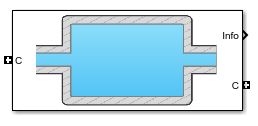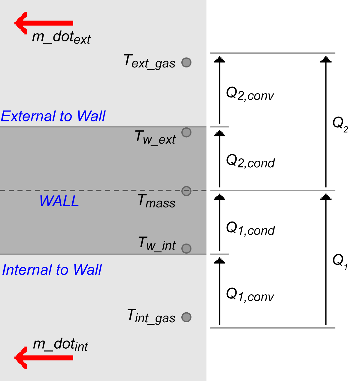# Control Volume System

Constant volume open thermodynamic system with heat transfer

•Libraries:
Powertrain Blockset / Propulsion / Combustion Engine Components / Fundamental Flow

## Description

The Control Volume System block models a constant volume open thermodynamic system with heat transfer. The block uses the conservation of mass and energy, assuming an ideal gas, to determine the pressure and temperature. The block implements an automotive-specific Constant Volume Pneumatic Chamber block that includes thermal effects related to the under hood of passenger vehicles. You can specify heat transfer models:

• Constant

• External input

• External wall convection

You can use the Control Volume System block to represent engine components that contain volume, including pipes and manifolds.

### Thermodynamics

The Control Volume System block implements a constant volume chamber containing an ideal gas. To determine the rate changes in temperature and pressure, the block uses the continuity equation and the first law of thermodynamics.

`$\begin{array}{l}\frac{d{T}_{vol}}{dt}=\frac{R{T}_{vol}}{{c}_{v}{V}_{ch}{P}_{vol}}\left(\sum \left({q}_{i}-{T}_{vol}{c}_{v}{\stackrel{˙}{m}}_{i}\right)-{Q}_{wall}\right)\\ \frac{d{P}_{vol}}{dt}=\frac{{P}_{vol}}{{T}_{vol}}\frac{d{T}_{vol}}{dt}+\frac{R{T}_{vol}}{{V}_{ch}}\sum {\stackrel{˙}{m}}_{i}\end{array}$`

The block uses this equation for the volume-specific enthalpy.

`${h}_{vol}={c}_{p}{T}_{vol}$`

The equations use these variables.

 ${\stackrel{˙}{m}}_{i}$ Mass flow rate at port qi Heat flow rate at port Vch Chamber volume Pvol Absolute pressure in the chamber R Ideal gas constant cv Specific heat at constant volume Tvol Absolute gas temperature Qwall Wall heat transfer rate hvol Volume-specific enthalpy cp Specific heat capacity

### Mass Fractions

The Control Volume Source block is part of a flow network. Blocks in the network determine the mass fractions that the block will track during simulation. The block can track these mass fractions:

• `O2` — Oxygen

• `N2` — Nitrogen

• `UnburnedFuel` — Unburned fuel

• `CO2` — Carbon dioxide

• `H2O` — Water

• `CO` — Carbon monoxide

• `NO` — Nitric oxide

• `NO2` — Nitrogen dioxide

• `PM` — Particulate matter

• `Air` — Air

• `BurnedGas` — Burned gas

Using the conservation of mass for each gas constituent, this equation determines the rate change:

`$\frac{d{y}_{vol,j}}{dt}=\frac{R{T}_{vol}}{{P}_{vol}{V}_{ch}}\left(\sum {\stackrel{˙}{m}}_{i}{y}_{i,j}+{y}_{vol,j}\sum {\stackrel{˙}{m}}_{i}\right)$`

The equations use these variables.

 Vch Chamber volume Pvol Absolute pressure in the chamber R Ideal gas constant Tvol Absolute gas temperature yi,j I-th port mass fraction for j = O2, N2, unburned fuel, CO2, H2O, CO, NO, NO2, PM, air, and burned gas yvol,j Control volume mass fraction for j = O2, N2, unburned fuel, CO2, H2O, CO, NO, NO2, PM, air, and burned gas ${\stackrel{˙}{m}}_{i}$ Mass flow rate for i = O2, N2, unburned fuel, CO2, H2O, CO, NO, NO2, PM, air, and burned gas

### External Wall Convection Heat Transfer Model

To calculate the heat transfer, you can configure the Control Volume Source block to calculate the heat transfer across the wall of the control volume.The block implements these equations to calculate the heat transfer, Q1, from the internal control volume gas to the internal wall depth, Dint_cond.

`${Q}_{1}={Q}_{1,conv}={Q}_{1,cond}$`

`${Q}_{1,conv}={h}_{int}\left({x}_{int}\right)•{A}_{int_conv}•\left({T}_{int_gas}-{T}_{w_int}\right)$`

`${Q}_{1,cond}={k}_{int}•\frac{{A}_{int_cond}}{{D}_{int_cond}}•\left({T}_{w_int}-{T}_{mass}\right)$`

The block implements these equations to calculate the heat transfer, Q2, from the external wall depth, Dext_cond to the external gas.

`${Q}_{2}={Q}_{2,conv}={h}_{ext}\left({x}_{ext}\right)•{A}_{ext_conv}•\left({T}_{w_ext}-{T}_{ext_gas}\right)$`

`${Q}_{2,cond}={k}_{ext}•\frac{{A}_{ext_cond}}{{D}_{ext_cond}}•\left({T}_{mass}-{T}_{w_ext}\right)$`

This equation expresses the heat stored in the thermal mass.

`$\frac{d{T}_{mass}}{dt}=\frac{{Q}_{1}-{Q}_{2}}{{c}_{{p}_{wall}}{m}_{wall}}$`

The block determines the interior convection heat transfer coefficient using a lookup table that is a function of the average mass flow rate.

`${\stackrel{˙}{m}}_{int_gas}=\frac{1}{2}\sum |{\stackrel{˙}{m}}_{i}|$`

The equations use these variables.

 Q1 Heat flow from the internal gas to a specified wall depth Q1,conv Heat flow convection from the internal gas to the internal wall Q1,cond Conduction heat transfer rate Q2 Heat transfer rate Q2,conv Convection heat transfer Q2,cond Heat flow conduction from the external middle portion of the wall to the external wall Qmass Heat stored in thermal mass hint Internal convection heat transfer coefficient xint Internal mass flow rate breakpoints Aint_conv Internal flow convection area Tint_gas Temperature of the gas inside the chamber Tw_int Temperature of the inside wall of the chamber kint Internal wall thermal conductivity Aint_cond Internal conduction area Dint_cond Internal wall thickness hext External convection heat transfer coefficient xext External velocity breakpoints Aext_conv External convection area Text_gas External gas temperature Tw_ext Temperature of the external wall of the chamber kext External wall thermal conductivity Aext_cond External conduction area Dext_cond External wall thickness Tmass Temperature of the thermal mass cp_wall Wall heat capacity mwall Thermal mass Flwspd External flow velocity ${\stackrel{˙}{m}}_{int_gas}$ Average internal mass flow rate

### Power Accounting

For the power accounting, the block implements these equation based on the number of inlet and outlet ports.

Bus Signal DescriptionEquations

`PwrInfo`

`PwrTrnsfrd` — Power transferred between blocks

• Positive signals indicate flow into block

• Negative signals indicate flow out of block

`PwrHeatFlwi`

Port `i` heat flow

qi

`PwrNotTrnsfrd` — Power crossing the block boundary, but not transferred

• Positive signals indicate an input

• Negative signals indicate a loss

`PwrHeatTrnsfr`

Heat transfer rate from wall to control volume

-Qwall

`PwrStored` — Stored energy rate of change

• Positive signals indicate an increase

• Negative signals indicate a decrease

`PwrHeatStored`

Rate of heat stored in the control volume

$\left({\sum }^{\text{​}}\left({q}_{i}\right)-{Q}_{wall}\right)$

For example, if you configure your block with 3 input ports and 2 outlet ports, the block implements these equations

Bus Signal DescriptionEquations

`PwrInfo`

`PwrTrnsfrd` — Power transferred between blocks

• Positive signals indicate flow into block

• Negative signals indicate flow out of block

`PwrHeatFlw1`

Inlet port 1 heat flow

q1

`PwrHeatFlw2`

Inlet port 2 heat flow

q2

`PwrHeatFlw3`

Inlet port 3 heat flow

q3

`PwrHeatFlw4`

Outlet port 4 heat flow

q4

`PwrHeatFlw5`

Outlet port 5 heat flow

q5

`PwrNotTrnsfrd` — Power crossing the block boundary, but not transferred

• Positive signals indicate an input

• Negative signals indicate a loss

`PwrHeatTrnsfr`

Heat transfer rate from wall to control volume

-Qwall

`PwrStored` — Stored energy rate of change

• Positive signals indicate an increase

• Negative signals indicate a decrease

`PwrHeatStored`

Rate of heat stored in the control volume

$\left({\sum }^{\text{​}}\left({q}_{i}\right)-{Q}_{wall}\right)$

## Ports

### Input

expand all

Bus containing:

• `MassFlw` — Mass flow rate through inlet, in kg/s

• `HeatFlw` — Inlet heat flow rate, in J/s

• `MassFrac` — Inlet mass fractions, dimensionless.

Specifically, a bus with these mass fractions:

• `O2MassFrac` — Oxygen

• `N2MassFrac` — Nitrogen

• `UnbrndFuelMassFrac` — Unburned fuel

• `CO2MassFrac` — Carbon dioxide

• `H2OMassFrac` — Water

• `COMassFrac` — Carbon monoxide

• `NOMassFrac` — Nitric oxide

• `NO2MassFrac` — Nitrogen dioxide

• `NOxMassFrac` — Nitric oxide and nitrogen dioxide

• `PmMassFrac` — Particulate matter

• `AirMassFrac` — Air

• `BrndGasMassFrac` — Burned gas

#### Dependencies

To create input ports, specify the Number of inlet ports parameter.

External heat transfer input to control volume, qhe, in Kg/s.

#### Dependencies

To create this port, select `External input` for the Heat transfer model parameter.

External flow velocity, Flwspd, in m/s.

#### Dependencies

To create this port, select `External wall convection` for the Heat transfer model parameter.

#### Dependencies

To create this port, select `External wall convection` for the Heat transfer model parameter.

### Output

expand all

Bus signal containing these block calculations.

SignalDescriptionUnits

`Vol`

`Prs`

Volume pressure

Pa

`Temp`

Volume temperature

K

`Enth`

Volume specific enthalpy

J/kg

`Species`

`O2MassFrac`

Oxygen mass fraction

NA

`N2MassFrac`

Nitrogen mass fraction

NA

`UnbrndFuelMassFrac`

Unburned gas mass fraction

NA

`CO2MassFrac`

Carbon dioxide mass fraction

NA

`H2OMassFrac`

Water mass fraction

NA

`COMassFrac`

Carbon monoxide mass fraction

NA

`NOMassFrac`

Nitric oxide mass fraction

NA

`NO2MassFrac`

Nitrogen dioxide mass fraction

NA

`NOxMassFrac`

Nitric oxide and nitrogen dioxide mass fraction

NA

`PmMassFrac`

Particulate matter mass fraction

NA

`AirMassFrac`

Air mass fraction

NA

`BrndGasMassFrac`

Burned gas mass fraction

NA

`HeatTrnsfr`

`HeatTrnsfrRate`

Wall heat transfer rate

J/s

`MassFlw`

Average internal mass flow rate

kg/s

`IntrnTemp`

Temperature of gas inside chamber

K

`PwrInfo`

`PwrTrnsfrd`

`PwrHeatFlwi`

Port `i` heat flow

W

`PwrNotTrnsfrd`

`PwrHeatTrnsfr`

Heat transfer rate from wall to control volume

W

`PwrStored`

`PwrHeatStored`

Rate of heat stored in the control volume

W

Bus containing the outlet control volume:

• `Prs` — Chamber pressure, in Pa

• `Temp` — Gas temperature, in K

• `Enth` — Specific enthalpy, in J/kg

• `MassFrac` — Mass fractions, dimensionless.

Specifically, a bus with these mass fractions:

• `O2MassFrac` — Oxygen

• `N2MassFrac` — Nitrogen

• `UnbrndFuelMassFrac` — Unburned fuel

• `CO2MassFrac` — Carbon dioxide

• `H2OMassFrac` — Water

• `COMassFrac` — Carbon monoxide

• `NOMassFrac` — Nitric oxide

• `NO2MassFrac` — Nitrogen dioxide

• `NOxMassFrac` — Nitric oxide and nitrogen dioxide

• `PmMassFrac` — Particulate matter

• `AirMassFrac` — Air

• `BrndGasMassFrac` — Burned gas

#### Dependencies

To create outlet ports, specify the Number of outlet ports parameter.

## Parameters

expand all

Block Options

Number of inlet ports.

#### Dependencies

To create inlet ports, specify the number.

Number of outlet ports.

#### Dependencies

To create outlet ports, specify the number.

#### Dependencies

Selecting `Constant` or ```External wall convection``` enables the Heat Transfer parameters.

Select color for block icon:

• `Cold` for blue

• `Hot` for red

General

Chamber volume, Vch, in m^3.

Initial chamber pressure, Pvol, in Pa.

Initial chamber temperature, Tvol, in K.

Ideal gas constant, R, in J/(kg*K).

Specific heat capacity, cp, in J/(kg·K).

Heat Transfer

Constant heat transfer rate, qhe, in J/s.

#### Dependencies

To enable this parameter, select `Constant` for the Heat transfer model parameter.

External convection heat transfer coefficient, hext, in W/(m^2K).

#### Dependencies

To enable this parameter, select ```External wall convection``` for the Heat transfer model parameter.

External velocity breakpoints, xext, in m/s.

#### Dependencies

To enable this parameter, select ```External wall convection``` for the Heat transfer model parameter.

External convection area, Aext_conv, in m^2.

#### Dependencies

To enable this parameter, select ```External wall convection``` for the Heat transfer model parameter.

Thermal mass, mwall, in kg.

#### Dependencies

To enable this parameter, select ```External wall convection``` for the Heat transfer model parameter.

Wall heat capacity, cp_wall, in J/(kg·K).

#### Dependencies

To enable this parameter, select ```External wall convection``` for the Heat transfer model parameter.

Initial mass temperature, Tmass, in K.

#### Dependencies

To enable this parameter, select ```External wall convection``` for the Heat transfer model parameter.

External wall thickness, Dext_cond, in m.

#### Dependencies

To enable this parameter, select ```External wall convection``` for the Heat transfer model parameter.

External conduction area, Aext_cond, in m^2.

#### Dependencies

To enable this parameter, select ```External wall convection``` for the Heat transfer model parameter.

External wall thermal conductivity, kext, in W/(m·K).

#### Dependencies

To enable this parameter, select ```External wall convection``` for the Heat transfer model parameter.

Internal wall thickness, Dint_cond, in m.

#### Dependencies

To enable this parameter, select ```External wall convection``` for the Heat transfer model parameter.

Internal conduction area, Aint_cond, in m^2.

#### Dependencies

To enable this parameter, select ```External wall convection``` for the Heat transfer model parameter.

Internal wall thermal conductivity, kint, in W/(m·K).

#### Dependencies

To enable this parameter, select ```External wall convection``` for the Heat transfer model parameter.

Internal convection heat transfer coefficient, hint, in W/(m^2K).

#### Dependencies

To enable this parameter, select ```External wall convection``` for the Heat transfer model parameter.

Internal velocity breakpoints, xint, in kg/s.

#### Dependencies

To enable this parameter, select ```External wall convection``` for the Heat transfer model parameter.

Internal convection area, Aint_conv, in m^2.

#### Dependencies

To enable this parameter, select ```External wall convection``` for the Heat transfer model parameter.

 Heywood, John B. Internal Combustion Engine Fundamentals. New York: McGraw-Hill, 1988.